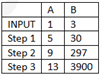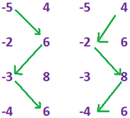# Basic Numeracy Solutions

The solutions to basic numeracy questions are given here. The solutions are detailed and easily understandable to help the CAT aspirants prepare for the exam more effectively. Check the questions and the solutions to analyse your current preparation level.

### Basic Numeracy- Solutions

1)Option (c)

Using Basic Remainder theorem

10/3 gives a remainder of 1. In fact, any power of 10 when divided by 3 gives a remainder of 1 (i.e. 10/3 remainder =1, 100/3 remainder =1 etc) Also, |Remainder= 1.Therefore individual remainders are 1 and 1 and 1-1=0. Answer is 0.

2) Option (c)

First write all the numbers in base 10

127 in base 8 = 7×1 + 2×8 + 1×82= 87 in base 10 12 in base 6 = 2×1+ 1×6= 8 in base 10

Adding both = 95 in base 10 Expressing this in base 5=340.

3) Option (c)

Converting base 2 to base 10 (11100)2=0+0+22+23+24=28

(1001)x=x0+x3=1+x3 Solving 1+x3 = 28 = 27+1= 33 +1, x=3.

4) Option (e)

Use the shortcut of answer options (Reverse Gear Approach) Answer is none of these , as none of the options a,b,c or d satisfy all three conditions.The number which satisfies these conditions is 11 5) option (d) (4x–1)2 = 42x– 2 If we further simplify we get the expression 42x/42

To solve this expression, all we need is to find the value of 42x Now let’s look back at our original equation.If 44x = 1600, we can find the value of 42x by taking the square root of both sides of the equation.Since the question asks for 42x/42 , the answer is 40/42 , which simplifies to 40/16 or 5/2. 6) HCF of 16 and x=4 .This implies that x has only two 2s in it HCF of 45 & x is 3 .This implies that x has only one 3 in it.

(a) 3: missing a 2

(b) 14: missing a 3

(c) 30: x cannot be divisible by 5

(d) 42: Correct. x and 210 are both divisible by 2 and 3

(e) 70: x cannot be divisible by 5 and must be divisible by 3.

6) Option (c)

Since a number= Quotient x Divisor + Remainder We can write the number as 48x +17.Therefore when this is divided by 12 we have 48x/12+17/12.From Basic Remainder theorem, we can see that the remainder is 5.

7) Option (d)

To find the maximum value of the expression, we need to find the minimum value of a,b and cThe minimum value that a,b and c can take is 1 each (since all are positive integers).Thus the minimum value of the expression is 0.

8) Option (b)

Using the shortcut of Assumption Assume any two digit number, say 10, then x=10

From Basic Remainder Theorem Remainder will be -1+0+1+4+3 =7.

9) AND 10)

P can only be 22,44,66 or 88

P+J=76 For an interval of 16 numbers, P can only take 44, then J =32

Similarly, L+O=74 O can only be 40, then L = 34, then K=30 Thus the range of numbers is between 30 and 44. Q=42 Also N/2 is odd,

therefore N= 38 and M= 36 Now the answers are direct.

9) Option (a)

10) Option (d)

11) Option (d)

Find the least value and the highest value of B/A Least B/A = 50/-4 = -12.5 Highest B/A = 50/1 = 50 Hence answer option d.

12) Option (b)

Observe the pattern 1 1 2 3 5 8 Every 3rd term is even.Hence a term which is a multiple of 3 will be even. 66 is a multiple of 3.Hence, the 66th term is even.

13) Option (c)

Tn= Tn-1 +Tn+1. 12th term= 144, 14th term = 12th term + 13th term 144+x= 377 x= 233 15th term = 13th term + 14th term = 233+ 377=610.

14) Option (d)

Coverting from base 2 to base 10 (100110110)2= 310 (no) (110010010)2= 402 (yes) (11001001)2 = 201 (yes).

15) Option (d)

Hence the machine undergoes 2 steps before it stops16) Option (a)

There are only 2 possibilities for the minimumminimum = -96 minimum = -72

Thus, the answer is option (a).

17) Option (c)

9/6remainder= 3 81/6remainder =3 729/6also remainder =3

In fact, any power of 9 when divided by 6, gives a remainder 3.

hence answer = 11 x 3/6(As there are 11 terms)= 33/6 = remainder =3.

18) Option (d)

Using the shortcuts of Assumption and Reverse Gear

The easiest way to solve this question is by assuming ‘N’ numbers which satisfy this question condition (i.e. their product should be unity)

Take 2 and 1/2 as the two positive numbers whose product is unity.

Here n=2. only option (c) and (d) are satisfied. The other 2 options can never be true. Take 4 positive numbers.

Let them be 1,1,1 and 1. Here n=4. option(c) is not true. This can never be the correct answer. Hence, by elimination, answer is option (d).

19) Option(c)

Using the Reverse Gear Approach Plug in values for “x” from the answer options If you just check for 981 and 982, only 6 will give a constant remainder. Hence, answer is option (c).

20) Option (d)

The easiest way to solve this question is to go from answer options.

Check each answer to find out which one satisfies the conditions mentioned in the question None of the options will give a product consisting entirely of 8s when multiplied by 13 Thus, answer is option.

21) option (a)

Let the number that Vineet want to multiply be ‘X’. He is expected to find the value of 45X. Instead, he found the value of 54X.The difference between the value that he got (54X) and what he was expected to get (45X), according to the question, is 540. i.e., 54X – 45X = 540 or (54 – 45) * X = 540 X = 60.Thus, the correct product = 45X = 45×60= 2700.

22) Option (e)

The distance between R and S is 713 – 712 = 712x 6 The distance between and two consecutive points is constant Value of Q = 712-6X712 = -5X712Value of P = -5×712-6×712 = -11×712 The correct answer is option (e).

23) Option (d)

57= 50+5+2= 3 coins 95= 50+25+10+10=4 coins 126=100+10+10+2+2+2=6 coins 27= 25+2= 2 coins Total =15.

24) Option (d)

Note that 7/8 remainder = 7 or -1 This means that any odd power of 7, when divided by 8; will give a remainder of -1 itself i.e.

((-1)7+ (-1)77+(-1)777…….)/8 = (-1-1-1-…)8 times = -8

The remainder when -8 is divided by 8, is 0

25) Option (b)

20 is equalized by 3 pairs of primes.(37,3) (29,11) and (23,17)

17 is equalized by 3 pairs of primes (31,3), (29,5) and (23,11)

18 is equalized by 4 pairs of primes (31,5) (29,7) (23,13) (19,17)

15 is equalized by 3 pairs of primes (23,7) (29,22) (17,13)

12 is equalized by 3 pairs of primes (19,5) (17,7) (13,11).

To get more such sample questions, visit CAT quantitative aptitude page. Stay tuned with BYJU’S to get notified about the latest CAT exam details along with the pattern, syllabus and previous year question papers.